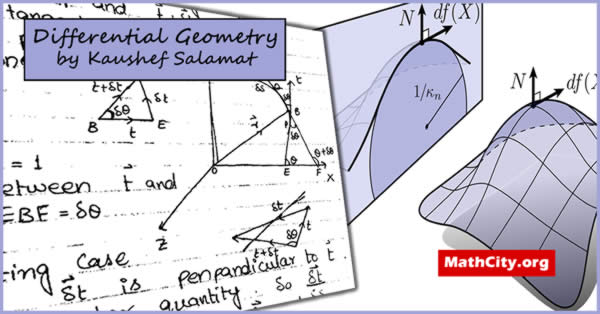# Differential Geometry (Notes) by Ms. Kaushef SalamatDifferential Geometry is an important field in the mathematical sciences. Usually, this is a compulsory or core subject for BS mathematics students.

These notes are send by Ms. Kaushef Salamat. We are really very thankful to her for providing these notes and appreciates her effort to publish these notes on MathCity.org

 Name Differential Geometry (Notes) Ms. Kaushef Salamat 241 pages PDF (see Software section for PDF Reader) 7.79 mB

In this section, the main heading are given below.

• Space curve
• Twisted curve
• Plane curve
• Tangent
• Arc
• Chord
• Arc length
• Equation of tangent
• Oscillating plane
• Principal normal
• Normal plane
• Curvature
• Equation of osculating plane
• Bi-normal vector
• Equation of Bi-normal
• Torsion
• Seret Frenet formulas
• Equation of tangent in Cartesian formulas
• Equation of osculating plane in Cartesian form
• Rectifying plane
• Circle of curvature
• Locus of centre of curvature
• The locus of centre of spherical curvature
• Fundamental theorem for space curves
• Intrinsic equation of a curve
• Spherical indicatrix of tangent
• Curvature and torsion of spherical indicatrix
• Spherical indicatrix of binormal of a curve
• The curvature and torsion of spherical indicatrix of binormal of a curve
• Spherical indicatrix of principal normal of a curve
• Skew curvature
• Tangent surface
• Involute
• Equation of involute
• Evolute of a curve
• Surface
• Equation of tangent plane to a surface
• Normal to a surface
• Curvature co-ordinates for a surface and parametric curves.
• Metric on a surface, first fundamental form for a surface, first order magnitude
• Directions on a surface
• Normal for a surface
• Second order magnitudes
• Weingarten equations
• Normal section of a surface at a point
• Meunier's theorem
• Normal curvature and radius of normal curvature
• Principal directions and principal curvature
• Differential equation for principal directions
• Euler's theorem
• Surface of revolution
• Normal surface
• One parameter family of surface*
• Characteristic of surface
• Envelop
• Edge of regression
• Equation of edge of regression
• Developable surfaces
• Geodesics or Geodesics line on a surface
• Geodesics curvature vector
• Geodesics curvature
• Differential equation for geodesics on a surface

• notes/differential-geometry-kaushef_salamat